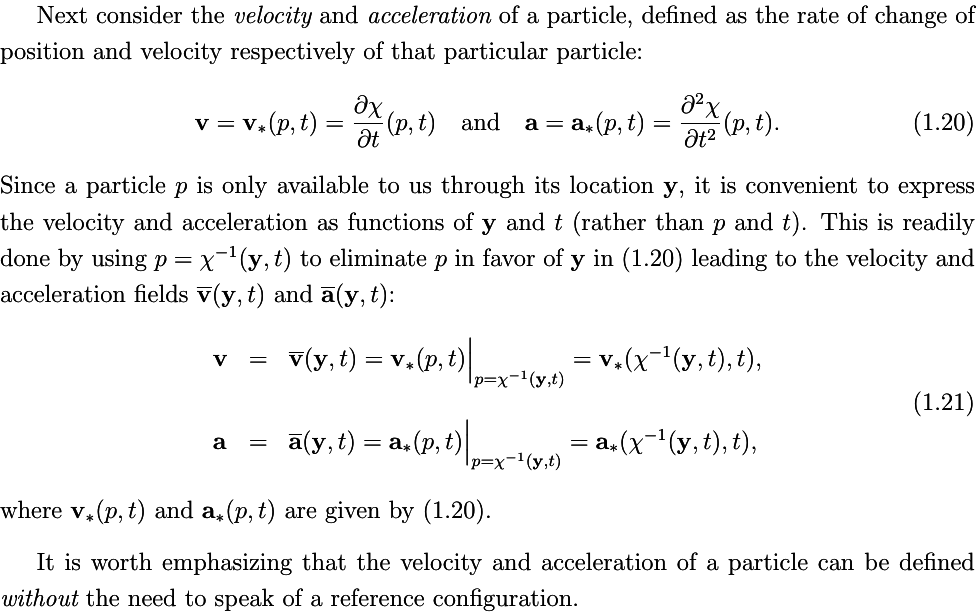# on 10-Jun-2020 (Wed)

#### Annotation 4730642369804

#body #configuration #continuum-mechanics #particle #position #region

We shall use the term “body” to be a mathematical abstraction of an “object that occurs in nature”. A body $$\mathcal B$$ is composed of a set of particles $$p$$ (or material points). In a given configuration of the body, each particle is located at some definite point $$\mathbf{y}$$ in three-dimensional space. The set of all the points in space, corresponding to the locations of all the particles, is the region $$\mathcal R$$ occupied by the body in that configuration. A particular body, composed of a particular set of particles, can adopt different configurations under the action of different stimuli (forces, heating etc.) and therefore occupy different regions of space under different conditions. Note the distinction between the body, a configuration of the body, and the region the body occupies in that configuration; we make these distinctions rigorous in what follows. Similarly note the distinction between a particle and the position in space it occupies in some configuration.

In order to appreciate the difference between a configuration and the region occupied in that configuration, consider the following example: suppose that a body, in a certain configuration, occupies a circular cylindrical region of space. If the object is “twisted” about its axis (as in torsion), it continues to occupy this same (circular cylindrical) region of space. Thus the region occupied by the body has not changed even though we would say that the body is in a different “configuration”.

#### pdf

cannot see any pdfs

#### Flashcard 5510566645004

Tags
#limitless
Question
Which are the technology villains?
Through our educational platform Kwik Learning, we have students in 195 countries and have generated tens of millions of podcast downloads. Our community has expressed a growing concern about their overreliance on technology and they come to us to upgrade their brains to find relief from these “four horsemen” of our age: digital deluge, digital distraction, digital dementia, and digital deduction. It’s important to note that overload, distraction, forgetfulness, and default thinking have been around for ages. While technology doesn’t cause these conditions, it has great potential to amplify them. The benefits of the digital age are plentiful, but let’s take a look at how the advances in technology that help you, can possibly also hinder you.

status measured difficulty not learned 37% [default] 0

#### Parent (intermediate) annotation

Open it
Through our educational platform Kwik Learning, we have students in 195 countries and have generated tens of millions of podcast downloads. Our community has expressed a growing concern about their overreliance on technology and they come to us to upgrade their brains to find relief from these “four horsemen” of our age: digital deluge, digital distraction, digital dementia, and digital deduction. It’s important to note that overload, distraction, forgetfulness, and default thinking have been around for ages. While technology doesn’t cause these conditions, it has great potential to amplify them. The benefits of the digital age are plentiful, but let’s take a look at how the advances in technology that help you, can possibly also hinder you.

#### Original toplevel document (pdf)

cannot see any pdfs

#### Flashcard 5510570839308

Tags
#limitless
Question
Describe the rat experiment and the relation between downtime and and memory. Which digital villain can be related to this experiment?

In a University of California, San Francisco, study on the effect of downtime, researchers gave rats a new experience and measured their brain waves during and after the activity. Under most circumstances, a new experience will express new neural activity and new neurons in the brain—that is, if the rat is allowed to have downtime. With downtime, the neurons made their way from the gateway of memory to the rest of the brain, where long-term memory is stored. The rats were able to record memories of their experiences, which is the basis for learning.

Doesn’t that make you wonder what happens if you don’t have downtime? There is a growing body of evidence that suggests that if we never let our mind wander or be bored for a moment, we pay a price—poor memory, mental fog, and fatigue.

status measured difficulty not learned 37% [default] 0

#### pdf

cannot see any pdfs

#### Flashcard 5525554728204

Question
Art. 96. A expressão "legislação tributária" compreende
[default - edit me]

status measured difficulty not learned 37% [default] 0

#### pdf

cannot see any pdfs

#### Flashcard 5525555776780

Question
[default - edit me]
as leis, os tratados e as convenções internacionais, os decretos e as normas complementares que versem, no todo ou em parte, sobre tributos e relações jurídicas a eles pertinentes.

status measured difficulty not learned 37% [default] 0

#### pdf

cannot see any pdfs

#### Flashcard 5525568097548

Tags
#continuum-mechanics
Question
When introducing the concepts of the continuum theory of materials, Rohan Abeyaratne encourages the reader to pay special attention to the distinctions between the different concepts introduced. List some of them.

The reader is encouraged to pay special attention to the distinctions between the different concepts introduced here. These concepts include the notions of

• a body,
• a configuration of the body,
• a reference configuration of the body,
• the region occupied by the body in some configuration,
• a particle (or material point),
• the location of a particle in some configuration,
• a deformation,
• a motion,
• Eulerian and Lagrangian descriptions of a physical quantity,
• Eulerian and Lagrangian spatial derivatives, and
• Eulerian and Lagrangian time derivatives (including the material time derivative).

status measured difficulty not learned 37% [default] 0

#### Parent (intermediate) annotation

Open it
The reader is encouraged to pay special attention to the distinctions between the different concepts introduced here. These concepts include the notions of a body, a configuration of the body, a reference configuration of the body, the region occupied by the body in some configuration, a particle (or material point), the location of a particle in some configuration, a deformation, a motion, Eulerian and Lagrangian descriptions of a physical quantity, Eulerian and Lagrangian spatial derivatives, and Eulerian and Lagrangian time derivatives (including the material time derivative).

#### Original toplevel document (pdf)

cannot see any pdfs

#### Flashcard 5525571505420

Tags
#body #configuration #continuum-mechanics #particle #position #region
Question
Introduce the concepts of body, particles, configuration and region regarding continuum mechanics.

We shall use the term “body” to be a mathematical abstraction of an “object that occurs in nature”. A body $$\mathcal B$$ is composed of a set of particles $$p$$ (or material points). In a given configuration of the body, each particle is located at some definite point $$\mathbf{y}$$ in three-dimensional space. The set of all the points in space, corresponding to the locations of all the particles, is the region $$\mathcal R$$ occupied by the body in that configuration. A particular body, composed of a particular set of particles, can adopt different configurations under the action of different stimuli (forces, heating etc.) and therefore occupy different regions of space under different conditions. Note the distinction between the body, a configuration of the body, and the region the body occupies in that configuration; we make these distinctions rigorous in what follows. Similarly note the distinction between a particle and the position in space it occupies in some configuration.

In order to appreciate the difference between a configuration and the region occupied in that configuration, consider the following example: suppose that a body, in a certain configuration, occupies a circular cylindrical region of space. If the object is “twisted” about its axis (as in torsion), it continues to occupy this same (circular cylindrical) region of space. Thus the region occupied by the body has not changed even though we would say that the body is in a different “configuration”.

status measured difficulty not learned 37% [default] 0

#### Parent (intermediate) annotation

Open it
We shall use the term “body” to be a mathematical abstraction of an “object that occurs in nature”. A body $$\mathcal B$$ is composed of a set of particles $$p$$ (or material points). In a given configuration of the body, each particle is located at some definite point $$\mathbf{y}$$ in three-dimensional space. The set of all the points in space, corresponding to the locations of all the particles, is the region $$\mathcal R$$ occupied by the body in that configuration. A particular body, composed of a particular set of particles, can adopt different configurations under the action of different stimuli (forces, heating etc.) and therefore occupy different regions of space under different conditions. Note the distinction between the body, a configuration of the body, and the region the body occupies in that configuration; we make these distinctions rigorous in what follows. Similarly note the distinction between a particle and the position in space it occupies in some configuration. In order to appreciate the difference between a configuration and the region occupied in that configuration, consider the following example: suppose that a body, in a certain configuration, occupies a circular cylindrical region of space. If the object is “twisted” about its axis (as in torsion), it continues to occupy this same (circular cylindrical) region of space. Thus the region occupied by the body has not changed even though we would say that the body is in a different “configuration”.

#### Original toplevel document (pdf)

cannot see any pdfs

#### Flashcard 5525573864716

[unknown IMAGE 4730646564108]
Tags
#continuum-mechanics #has-images
Question
Define body, region, particle and configuration the body "more formally".

More formally, in continuum mechanics a body $$\mathcal B$$ is a collection of elements which can be put into one-to-one correspondence with some region $$\mathcal R$$ of Euclidean point space. An element $$p \in \mathcal B$$ is called a particle (or material point). Thus, given a body $$\mathcal B$$, there is necessarily a mapping $$\chi$$ that takes particles $$p \in \mathcal B$$ into their geometric locations $$y \in \mathcal R$$ in three-dimensional Euclidean space:

$$y = \chi(p) \quad \textrm{where} \quad p \in \mathcal B, \mathbf{y} \in \mathcal R.$$

The mapping $$\chi$$ is called a configuration of the body $$\mathcal B$$; $$\mathbf y$$ is the position occupied by the particle $$p$$ in the configuration $$\chi$$; and $$\mathcal R$$ is the region occupied by the body in the configuration $$\chi$$. Often, we write $$\mathcal R = \chi \left( \mathcal B \right)$$.

status measured difficulty not learned 37% [default] 0

#### Parent (intermediate) annotation

Open it
More formally, in continuum mechanics a body $$\mathcal B$$ is a collection of elements which can be put into one-to-one correspondence with some region $$\mathcal R$$ of Euclidean point space. An element $$p \in \mathcal B$$ is called a particle (or material point). Thus, given a body $$\mathcal B$$, there is necessarily a mapping $$\chi$$ that takes particles $$p \in \mathcal B$$ into their geometric locations $$y \in \mathcal R$$ in three-dimensional Euclidean space: $$y = \chi(p) \quad \textrm{where} \quad p \in \mathcal B, \mathbf{y} \in \mathcal R.$$ The mapping $$\chi$$ is called a configuration of the body $$\mathcal B$$; $$\mathbf y$$ is the position occupied by the particle $$p$$ in the configuration $$\chi$$; and $$\mathcal R$$ is the region occupied by the body in the configuration $$\chi$$. Often, we write $$\mathcal R = \chi \left( \mathcal B \right)$$.

#### Original toplevel document (pdf)

cannot see any pdfs

#### Flashcard 5525576748300

Tags
#continuum-mechanics #reference-configuration
Question
Describe two reasons considering a reference configuration.

In order to identify a particle of a body, we must label the particles. The abstract particle label $$p$$, while perfectly acceptable in principle and intuitively clear, is not convenient for carrying out calculations. It is more convenient to pick some arbitrary configuration of the body, say $$\chi_\textrm{ref}$$ , and use the (unique) position $$x = \chi_\textrm{ref} (p)$$ of a particle in that configuration to label it instead. Such a configuration $$\chi_\textrm{ref} (p)$$ is called a reference configuration of the body. It simply provides a convenient way in which to label the particles of a body. The particles are now labeled by $$\mathbf x$$ instead of $$p$$.

A second reason for considering a reference configuration is the following: we can study the geometric characteristics of a configuration $$\chi$$ by studying the geometric properties of the points occupying the region $$\mathcal R = \chi (\mathcal B)$$. This is adequate for modeling certain materials (such as many fluids) where the behavior of the material depends only on the characteristics of the configuration currently occupied by the body. In describing most solids however one often needs to know the changes in geometric characteristics between one configuration and another configuration (e.g. the change in length, the change in angle etc.). In order to describe the change in a geometric quantity one must necessarily consider (at least) two configurations of the body: the configuration that one wishes to analyze, and a reference configuration relative to which the changes are to be measured.

status measured difficulty not learned 37% [default] 0

#### pdf

cannot see any pdfs

#### Flashcard 5525582253324

[unknown IMAGE 5525580942604]
Tags
#continuum-mechanics #has-images
Question
Draw a scheme showing a body, a reference configuration and another configuration, as well as the mapping between a particle in the body and the configurations.

status measured difficulty not learned 37% [default] 0

#### pdf

cannot see any pdfs

#### Annotation 5525586971916

#continuum-mechanics #deformation

This induces a mapping $$\mathbf y = \hat{\mathbf y} (\mathbf x)$$ from $$\mathcal R_\textrm{ref} \rightarrow \mathcal R$$:

$$\mathbf y = \hat{\mathbf y} (\mathbf x) \stackrel{\textrm{def}}{=} \chi \left( \chi_\textrm{ref}^{-1} \left( \mathbf x\right) \right), \qquad \mathbf x \in \mathcal R_\textrm{ref}, \mathbf y \in \mathcal R;$$

$$\hat{\mathbf y}$$ is called a deformation of the body from the reference confinguration $$\chi_\textrm{ref}$$.

#### pdf

cannot see any pdfs

#### Flashcard 5525590117644

Tags
#continuum-mechanics #deformation
Question

This induces a mapping $$\mathbf y = \hat{\mathbf y} (\mathbf x)$$ from $$\mathcal R_\textrm{ref} \rightarrow \mathcal R$$:

$$\mathbf y = \hat{\mathbf y} (\mathbf x) \stackrel{\textrm{def}}{=} \chi \left( \chi_\textrm{ref}^{-1} \left( \mathbf x\right) \right), \qquad \mathbf x \in \mathcal R_\textrm{ref}, \mathbf y \in \mathcal R;$$

$$\hat{\mathbf y}$$ is called a [...] of the body from the reference confinguration $$\chi_\textrm{ref}$$.

deformation

status measured difficulty not learned 37% [default] 0

#### Parent (intermediate) annotation

Open it
el{\textrm{def}}{=} \chi \left( \chi_\textrm{ref}^{-1} \left( \mathbf x\right) \right), \qquad \mathbf x \in \mathcal R_\textrm{ref}, \mathbf y \in \mathcal R;\) $$\hat{\mathbf y}$$ is called a <span>deformation of the body from the reference confinguration $$\chi_\textrm{ref}$$. <span>

#### Original toplevel document (pdf)

cannot see any pdfs

#### Flashcard 5525591690508

[unknown IMAGE 5525580942604]
Tags
#continuum-mechanics #has-images
Question
Describe some simplifications which arise when considering a single fixed reference configuration.
When working with a single fixed reference configuration, as we will most often do, one can dispense with talking about the body $$\mathcal B$$, a configuration $$\chi$$ and the particle $$p$$, and work directly with the region $$\mathcal R_\textrm{ref}$$, the deformation $$\mathbf y \left( \mathbf x \right)$$ and the position $$\mathbf x$$.

status measured difficulty not learned 37% [default] 0

#### pdf

cannot see any pdfs

#### Flashcard 5525597195532

Tags
#continuum-mechanics #has-images
Question
Discuss about material description, Eulerian or spatial description and Lagrangian or referential description.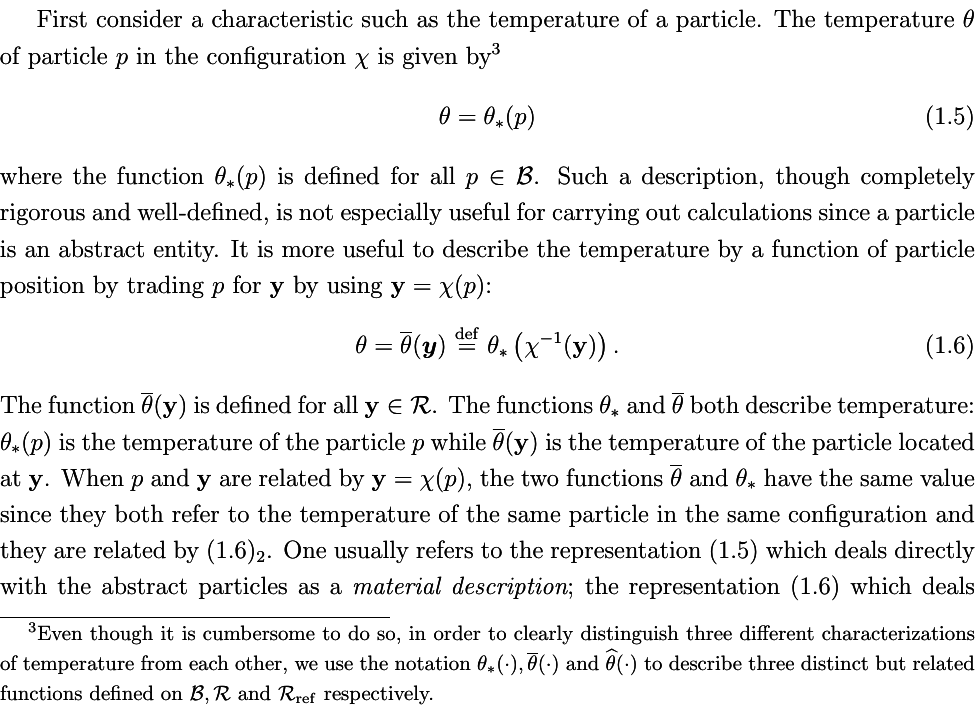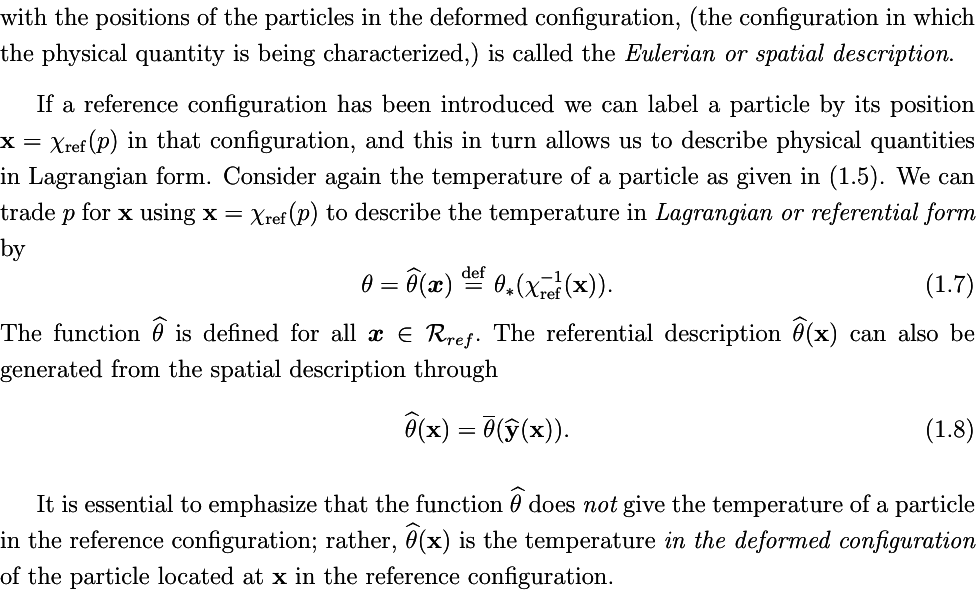status measured difficulty not learned 37% [default] 0

#### pdf

cannot see any pdfs

#### Flashcard 5525602700556

Tags
#continuum-mechanics #has-images
Question
Discuss about (differentiate) Cauchy and first Piola-Kirchhoff stress tensors.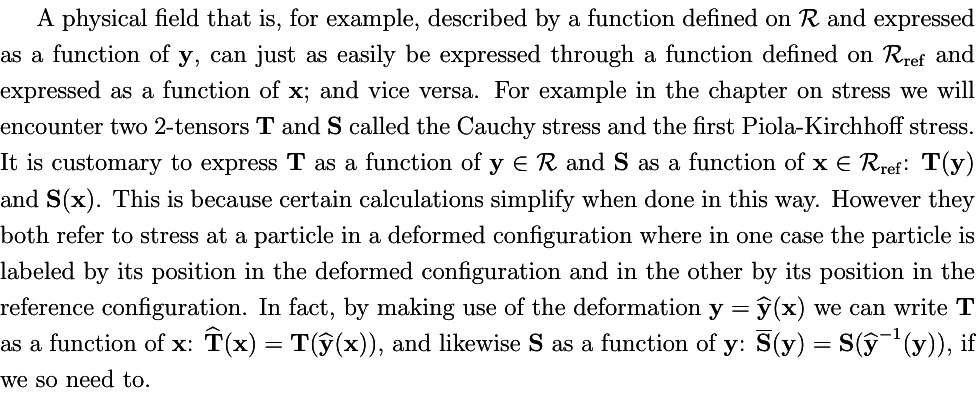status measured difficulty not learned 37% [default] 0

#### pdf

cannot see any pdfs

#### Flashcard 5525606632716

Tags
#continuum-mechanics #has-images
Question
Explain the reason for using Grad, grad, Div, div, Curl, and curl as operator names in continuum mechanics.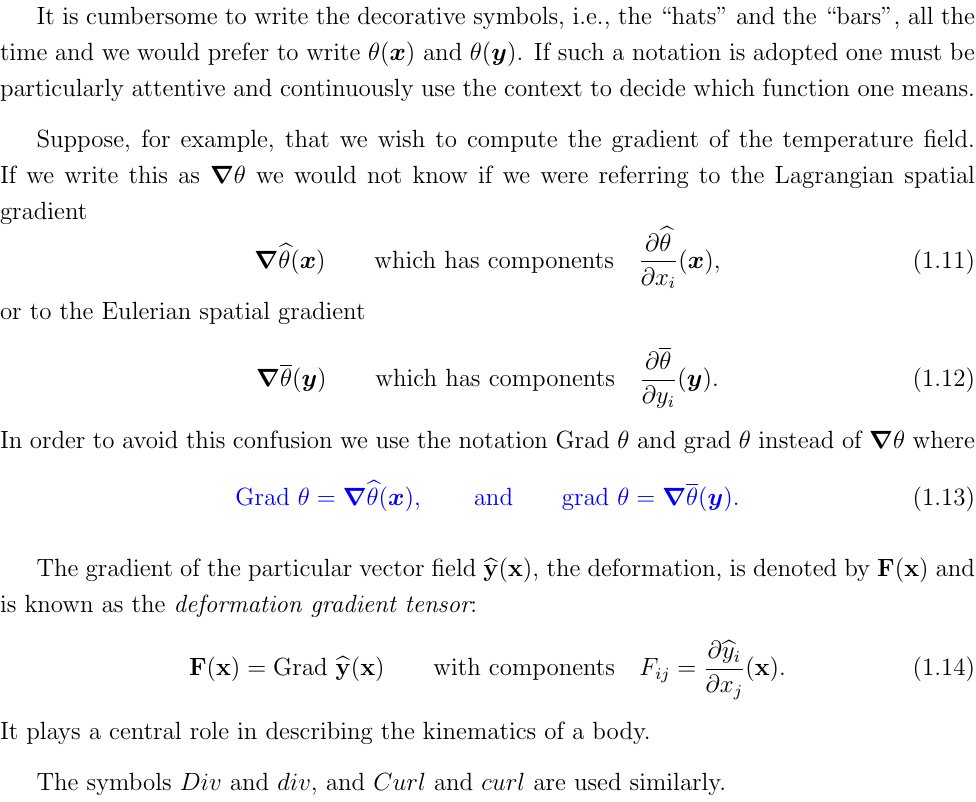status measured difficulty not learned 37% [default] 0

#### pdf

cannot see any pdfs

#### Flashcard 5525610827020

Tags
#continuum-mechanics #has-images
Question
Derive the relationship between $$\operatorname{Grad} \theta$$ and $$\operatorname{grad} \theta$$.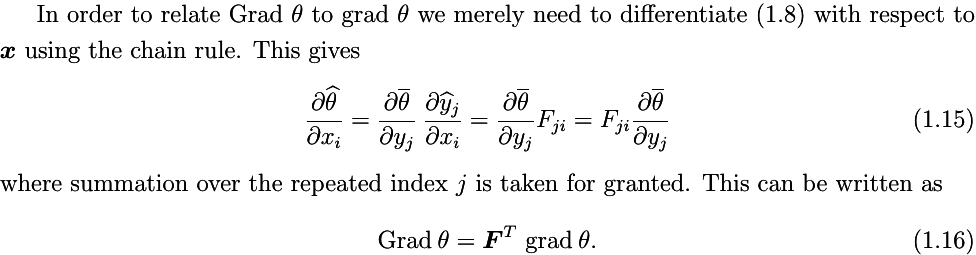status measured difficulty not learned 37% [default] 0

#### pdf

cannot see any pdfs

#### Flashcard 5525614234892

Tags
#continuum-mechanics #has-images
Question
Describe the motion of a body.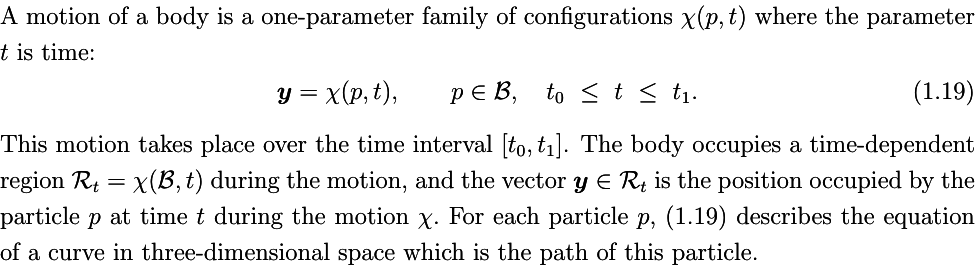status measured difficulty not learned 37% [default] 0

#### pdf

cannot see any pdfs

#### Flashcard 5525620526348

Tags
#continuum-mechanics #has-images
Question
Define the velocity and acceleration of a particle in the context of continuum mechanics.